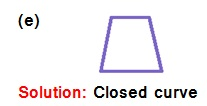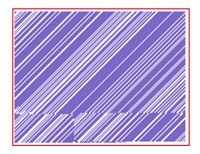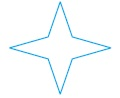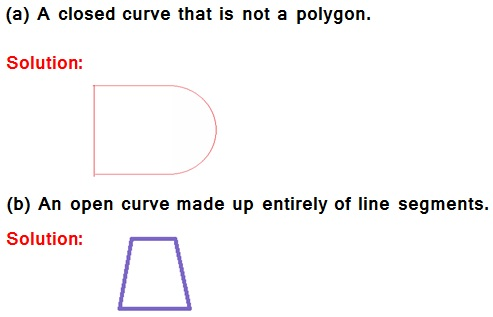Study Materials: ncert solutions

Our ncert solutions for Exercise 4.2 Class 6 maths 4. Basic Geometrical Ideas - ncert solutions - Toppers Study is the best material for English Medium students cbse board and other state boards students.

Solutions ⇒ Class 6th ⇒ Mathematics ⇒ 4. Basic Geometrical Ideas

# Exercise 4.2 Class 6 maths 4. Basic Geometrical Ideas - ncert solutions - Toppers Study

Topper Study classes prepares ncert solutions on practical base problems and comes out with the best result that helps the students and teachers as well as tutors and so many ecademic coaching classes that they need in practical life. Our ncert solutions for Exercise 4.2 Class 6 maths 4. Basic Geometrical Ideas - ncert solutions - Toppers Study is the best material for English Medium students cbse board and other state boards students.

## Exercise 4.2 Class 6 maths 4. Basic Geometrical Ideas - ncert solutions - Toppers Study

CBSE board students who preparing for class 6 ncert solutions maths and Mathematics solved exercise chapter 4. Basic Geometrical Ideas available and this helps in upcoming exams 2023-2024.

### You can Find Mathematics solution Class 6 Chapter 4. Basic Geometrical Ideas

• All Chapter review quick revision notes for chapter 4. Basic Geometrical Ideas Class 6
• NCERT Solutions And Textual questions Answers Class 6 Mathematics
• Extra NCERT Book questions Answers Class 6 Mathematics
• Importatnt key points with additional Assignment and questions bank solved.

NCERT Solutions do not only help you to cover your syllabus but also will give to textual support in exams 2023-2024 to complete exercise 4.2 maths class 6 chapter 4 in english medium. So revise and practice these all cbse study materials like class 6 maths chapter 4.2 in english ncert book. Also ensure to repractice all syllabus within time or before board exams for ncert class 6 maths ex 4.2 in english.

See all solutions for class 6 maths chapter 4 exercise 4 in english medium solved questions with answers.

### Exercise 4.2 class 6 Mathematics Chapter 4. Basic Geometrical Ideas

• Exercise 4.2 Class 6 Maths 4. Basic Geometrical Ideas - Ncert Solutions - Toppers Study
• Class 6 Ncert Solutions
• Solution Chapter 4. Basic Geometrical Ideas Class 6
• Solutions Class 6
• Chapter 4. Basic Geometrical Ideas Exercise 4.2 Class 6

## Exercise 4.2 Class 6 maths 4. Basic Geometrical Ideas - ncert solutions - Toppers Study

Exercise 4.2

Q1. Classify the following curves as (i) Open or (ii) Closed.Solution:

(i) Open curves : (a) and (c)

(ii) Close curves : (b) (d) and (e)

Q2. Draw rough diagrams to illustrate the following :
(a) Open curve (b) Closed curve.

Solution:Q3. Draw any polygon and shade its interior.

Solution:Q4. Consider the given figure and answer the questions :
(a) Is it a curve? (b) Is it closed?Solution:

(a) Is it a curve?

Solution: yes, it is a curve

(b) Is it closed?

Solution: yes, it is a closed

Q5. Illustrate, if possible, each one of the following with a rough diagram:
(a) A closed curve that is not a polygon.
(b) An open curve made up entirely of line segments.
(c) A polygon with two sides.

Solution:(c) A polygon with two sides.

Solution:  The polygon can be made with three or more sides. There is no polygon with two sides.

Important Study materials for classes 06, 07, 08,09,10, 11 and 12. Like CBSE Notes, Notes for Science, Notes for maths, Notes for Social Science, Notes for Accountancy, Notes for Economics, Notes for political Science, Noes for History, Notes For Bussiness Study, Physical Educations, Sample Papers, Test Papers, Mock Test Papers, Support Materials and Books.Mathematics Class - 11th

NCERT Maths book for CBSE Students.

books

## Study Materials List:

##### Solutions ⇒ Class 6th ⇒ Mathematics
1. Knowing Our Numbers
2. Whole Numbers
3. Playing with Numbers
4. Basic Geometrical Ideas
5. Understanding Elementary Shapes
6. Integers
7. Fractions
8. Decimals

New Books